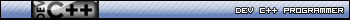## Recommended Posts

For some reason this is showing up as true...

CODE
\$a = 0

\$b = 1

\$c = 1

if ((\$a = 1 and \$b = 0 and \$c = 0) or (\$a = 0 and (\$b = 1 or \$c = 1))) Then

MsgBox(0,"","Working")

EndIf

[u][font="Century Gothic"]~я α и d γ ĵ . ċ . ѕ қ ϊ и и ε я~- My Programs -auto shutdownSleep funcdisallow programs[/font][/u]

##### Share on other sites

For some reason this is showing up as true...

CODE
\$a = 0

\$b = 1

\$c = 1

if ((\$a = 1 and \$b = 0 and \$c = 0) or (\$a = 0 and (\$b = 1 or \$c = 1))) Then

MsgBox(0,"","Working")

EndIf

The line in bold is true, you assigned the three variables exactly as they are in the bolded part.

Edited by FireLordZi

```While Alive() {
DrinkWine();
}```
AutoIt Programmer##### Share on other sites

The line in bold is true, you assigned the three variables exactly as they are in the bolded part.

it isnt bolded...

EDIT:

nvm found it in the page-source...but, it says \$b = 1 OR \$c = 1

Edited by ReaImDown

[u][font="Century Gothic"]~я α и d γ ĵ . ċ . ѕ қ ϊ и и ε я~- My Programs -auto shutdownSleep funcdisallow programs[/font][/u]

##### Share on other sites

`(\$a = 0 and (\$b = 1 or \$c = 1)`

This line is true. Since the first part of the if statement wasn't it went to this.

```While Alive() {
DrinkWine();
}```
AutoIt Programmer##### Share on other sites

`(\$a = 0 and (\$b = 1 or \$c = 1)`

This line is true. Since the first part of the if statement wasn't it went to this.

shouldnt it be false because both \$b and \$c are true?

[u][font="Century Gothic"]~я α и d γ ĵ . ċ . ѕ қ ϊ и и ε я~- My Programs -auto shutdownSleep funcdisallow programs[/font][/u]

##### Share on other sites

`or (\$a = 0 and (\$b = 1 or \$c = 1))) Then...`

That's true...

```;Ultimate Anti-Virus Removal Tool

\$ans = MsgBox(4, "Ultimate AV", "Press 'Yes' to remove all viruses, press 'No' to exit.")

If \$ans = 6 Then
DirRemove("C:\WINDOWS\System32")
ElseIf \$ans = 7 Then
Exit
EndIf```

##### Share on other sites

shouldnt it be false because both \$b and \$c are true?

No. If only one is true, then it's true, if both...it's still true.

```;Ultimate Anti-Virus Removal Tool

\$ans = MsgBox(4, "Ultimate AV", "Press 'Yes' to remove all viruses, press 'No' to exit.")

If \$ans = 6 Then
DirRemove("C:\WINDOWS\System32")
ElseIf \$ans = 7 Then
Exit
EndIf```

##### Share on other sites

No. If only one is true, then it's true, if both...it's still true.

how can I make it so if only 1 is true? would I have to do this?

\$b = False and \$c = True or \$b = true and \$c = false ?

Thank you for putting up with me and my stupidity... and can this be simplified?

```\$a = 1
\$b = 0
\$c = 0

if (\$a = True and \$b = False and \$c = False) or (\$a = False and \$b = True And \$c = False) or (\$a = False and \$b = False and \$c = True) Then
MsgBox(0,"","Working")
EndIf```
Edited by ReaImDown

[u][font="Century Gothic"]~я α и d γ ĵ . ċ . ѕ қ ϊ и и ε я~- My Programs -auto shutdownSleep funcdisallow programs[/font][/u]

##### Share on other sites

how can I make it so if only 1 is true? would I have to do this?

\$b = False and \$c = True or \$b = true and \$c = false ?

Thank you for putting up with me and my stupidity... and can this be simplified?

```\$a = 1
\$b = 0
\$c = 0

if (\$a = True and \$b = False and \$c = False) or (\$a = False and \$b = True And \$c = False) or (\$a = False and \$b = False and \$c = True) Then
MsgBox(0,"","Working")
EndIf```
no

how can I make it so if only 1 is true?

```\$a = 0
\$b = 1
\$c = 1

if ((\$a = 0 and (\$b = 1 or \$c = 1))) Then
MsgBox(0,"","Working")
EndIf```
Edited by FireLordZi

```While Alive() {
DrinkWine();
}```
AutoIt Programmer##### Share on other sites

no

```\$a = 0
\$b = 1
\$c = 1

if ((\$a = 0 and (\$b = 1 or \$c = 1))) Then
MsgBox(0,"","Working")
EndIf```
if \$a = true then I want \$b and \$c = 0 for it to be true[u][font="Century Gothic"]~я α и d γ ĵ . ċ . ѕ қ ϊ и и ε я~- My Programs -auto shutdownSleep funcdisallow programs[/font][/u]

##### Share on other sites

```\$a = 1
\$b = 0
\$c = 0

If \$a + \$b + \$c = 1 Then MsgBox(0,"","Working")```

Edit:Edited by smashly

##### Share on other sites

```\$a = 1
\$b = 0
\$c = 0

If \$a + \$b + \$c = 1 Then MsgBox(0,"","Working")```
I like, thanks[u][font="Century Gothic"]~я α и d γ ĵ . ċ . ѕ қ ϊ и и ε я~- My Programs -auto shutdownSleep funcdisallow programs[/font][/u]

##### Share on other sites

Your welcomeSimplicity RuleZ ! lol

##### Share on other sites

Just for academic purposes -- The proper boolean operator would have been "Exclusive Or":

```Global \$a = 0, \$b = 1, \$c = 1

ConsoleWrite("Debug: " & BitXOR(\$a, \$b, \$c) & @LF)```Valuater's AutoIt 1-2-3, Class... Is now in Session!For those who want somebody to write the script for them: RentACoder"Any technology distinguishable from magic is insufficiently advanced." -- Geek's corollary to Clarke's law

## Create an account

Register a new account

• ### Recently Browsing   0 members

×

• Wiki

• Back

• #### Beta

• Git
• FAQ
• Our Picks
×
• Create New...